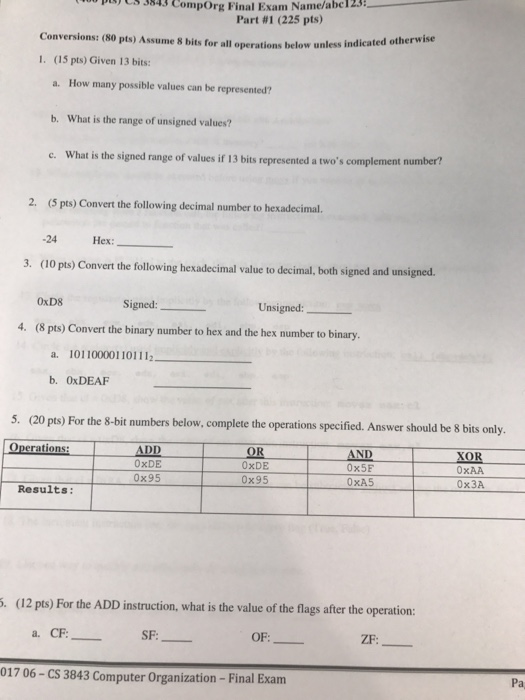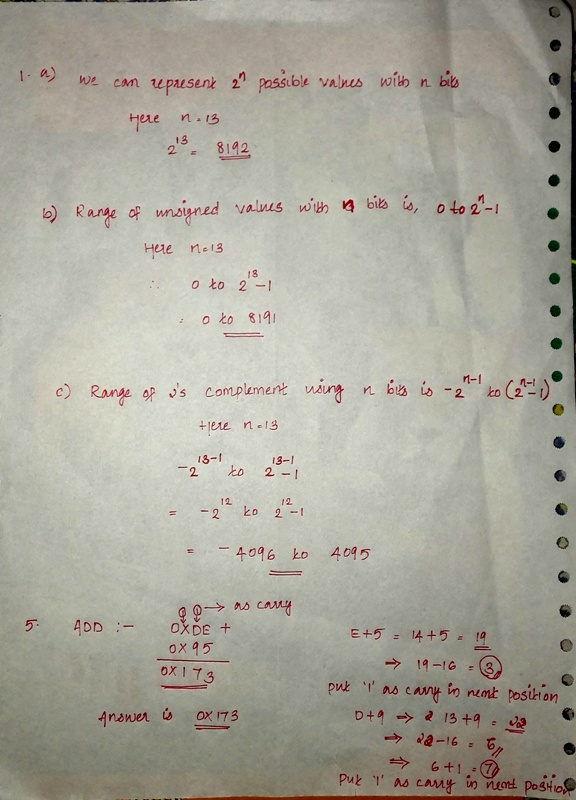# Question & Answer: Given 13 bits: a. How many possible values can be represented? b. What is the range of u…..Help on 1 & 5

Given 13 bits: a. How many possible values can be represented? b. What is the range of unsigned values? c. What is the signed range of values if 13 bits represented a two’s complement number? Convert the following decimal number to hexadecimal. -24 Hex: ____ Convert the following hexadecimal value to decimal, both signed and unsigned. 0 times D8 Signed: _____ Unsigned: _____ Convert the binary number to hex and the hex number to binary. a. 10110000110111_2 _____ b. 0 times DEAF _____ For the 8-bit numbers below, complete the operations specified. Answer should be 8 bits only. For the ADD instruction, what is the value of the flags after the operation: a. CF: _____ SF: _____ OF: _____ ZF: _____